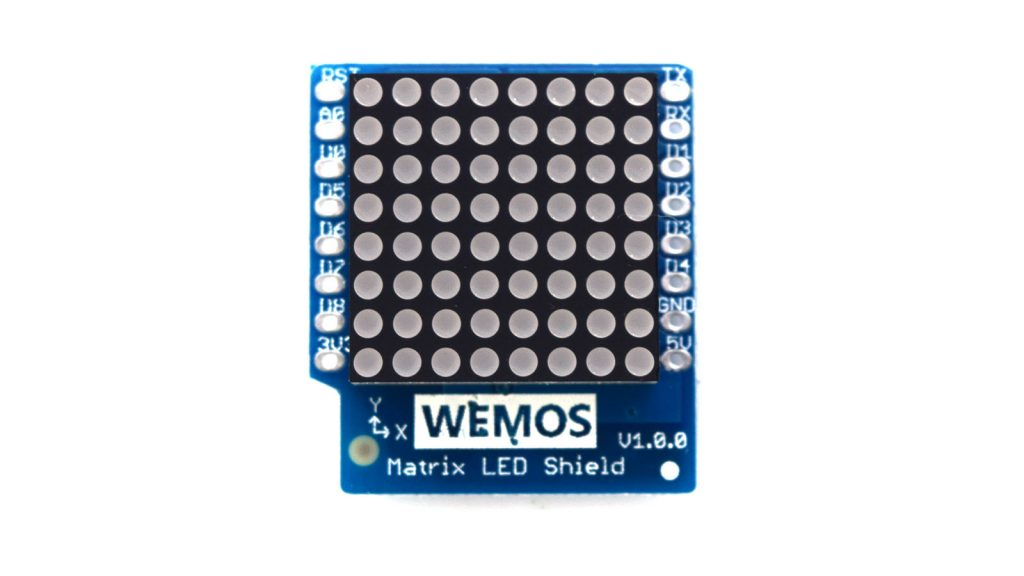# MH ET LIVE ESP32 MINI KIT and Matrix LED shield example

A new shield for the Wemos Mini came my way recently, this is called the MATRIX LED Shield – You can find out more at https://wiki.wemos.cc/products:d1_mini_shields:matrix_led_shieldThe board uses a TM1640, as you can see there are 8×8 LEDs with 8 levels of intensity. The shield will also use the D5 an D7 pins of your wemos mini, so these won’t be available for any other projects

In this example we will once again connect it to the MH ET LIVE ESP32 MINI KIT

Code

This requires the library from wemos to be slightly modified – WEMOS_Matrix_LED_Shield_Arduino_Library-master

The details are as follows, the original line in WEMOS_Matrix_LED.h , on the MH ET LIVE ESP32 MINI KIT board you need to change the pins, this is not difficult to-do. The attached library has this already done but if you want to do this yourself you need to make the following change

//MLED(uint8_t _intensity=4, byte dataPin=D7, byte clockPin=D5);
MLED(uint8_t _intensity=4, byte dataPin=23, byte clockPin=18);

There are a couple of built in examples but here are a few examples that will flash various LEDs

```#include <WEMOS_Matrix_LED.h>

MLED mled(5); //set intensity=5

void setup()
{

}

void loop() {

for(int y=0;y<8;y++)
{
for(int x=0;x<8;x++)
{
mled.dot(x,y); // draw dot
mled.display();
delay(200);
}
}
}
```

And another example

```#include <WEMOS_Matrix_LED.h>

MLED mled(5); //set intensity=5

void setup()
{

}

void loop() {

for(int y=0;y<8;y++)
{
mled.dot(0,y); // draw dot
mled.display();
delay(200);
}
}
[/codesyntax]

The next example

[cpp]
#include <WEMOS_Matrix_LED.h>

MLED mled(5); //set intensity=5
int randX, randY;

void setup()
{
Serial.begin(9600);

}

void loop()
{
randX = random(8);
randY = random(8);
mled.dot(randX,randY); // draw dot
mled.display();
delay(200);
}
```

and yet another example

```#include <WEMOS_Matrix_LED.h>

MLED mled(5); //set intensity=5
int randX, randY;

void setup()
{
Serial.begin(9600);

}

void loop()
{
randX = random(8);
randY = random(8);
mled.dot(randX,randY); // draw dot
mled.display();
delay(200);
mled.dot(randX,randY,0);//clear dot
mled.display();
delay(200);
}

```